# Introducing {workboots}

Generate bootstrap prediction intervals from a tidymodel workflow!
rstats
tidymodels
workboots
Author

Mark Rieke

Published

March 14, 2022

Code
``````# setup themes
ggplot2::theme_set(
ggplot2::theme_minimal(base_family = "Roboto Slab",
base_size = 14) +
ggplot2::theme(plot.title.position = "plot",
plot.background = ggplot2::element_rect(fill = "white", color = "white"))
)``````

Sometimes, we want a model that generates a range of possible outcomes around each prediction and may opt for a model that can generate a prediction interval, like a linear model. Other times, we just care about point predictions and may opt to use a more powerful model like XGBoost. But what if we want the best of both worlds: getting a range of predictions while still using a powerful model? That’s where `{workboots}` comes to the rescue! `{workboots}` uses bootstrap resampling to train many models which can be used to generate a range of outcomes — regardless of model type.# Installation

Version 0.1.0 of `{workboots}` is available on CRAN. Given that the package is still in early development, however, I’d recommend installing the development version from github:

Code
``````# install from CRAN
install.packages("workboots")

# or install the development version
devtools::install_github("markjrieke/workboots")``````

# Usage

`{workboots}` builds on top of the `{tidymodels}` suite of packages and is intended to be used in conjunction with a tidymodel workflow. Teaching how to use `{tidymodels}` is beyond the scope of this post, but some helpful resources are linked at the bottom for further exploration.

We’ll walk through two examples that show the benefit of the package: estimating a linear model’s prediction interval and generating a prediction interval for a boosted tree model.

## Estimating a prediction interval

Let’s get started with a model we know can generate a prediction interval: a basic linear model. In this example, we’ll use the Ames housing dataset to predict a home’s price based on its square footage.

Code
``````library(tidymodels)

# setup our data
data("ames")
ames_mod <- ames %>% select(First_Flr_SF, Sale_Price)``````
Code
``````# relationship between square footage and price
ames_mod %>%
ggplot(aes(x = First_Flr_SF, y = Sale_Price)) +
geom_point(alpha = 0.25) +
scale_y_continuous(labels = scales::dollar_format(), trans = "log10") +
scale_x_continuous(labels = scales::comma_format(), trans = "log10") +
labs(title = "Relationship between Square Feet and Sale Price",
subtitle = "Linear relationship between the log transforms of square footage and price",
x = NULL,
y = NULL)``````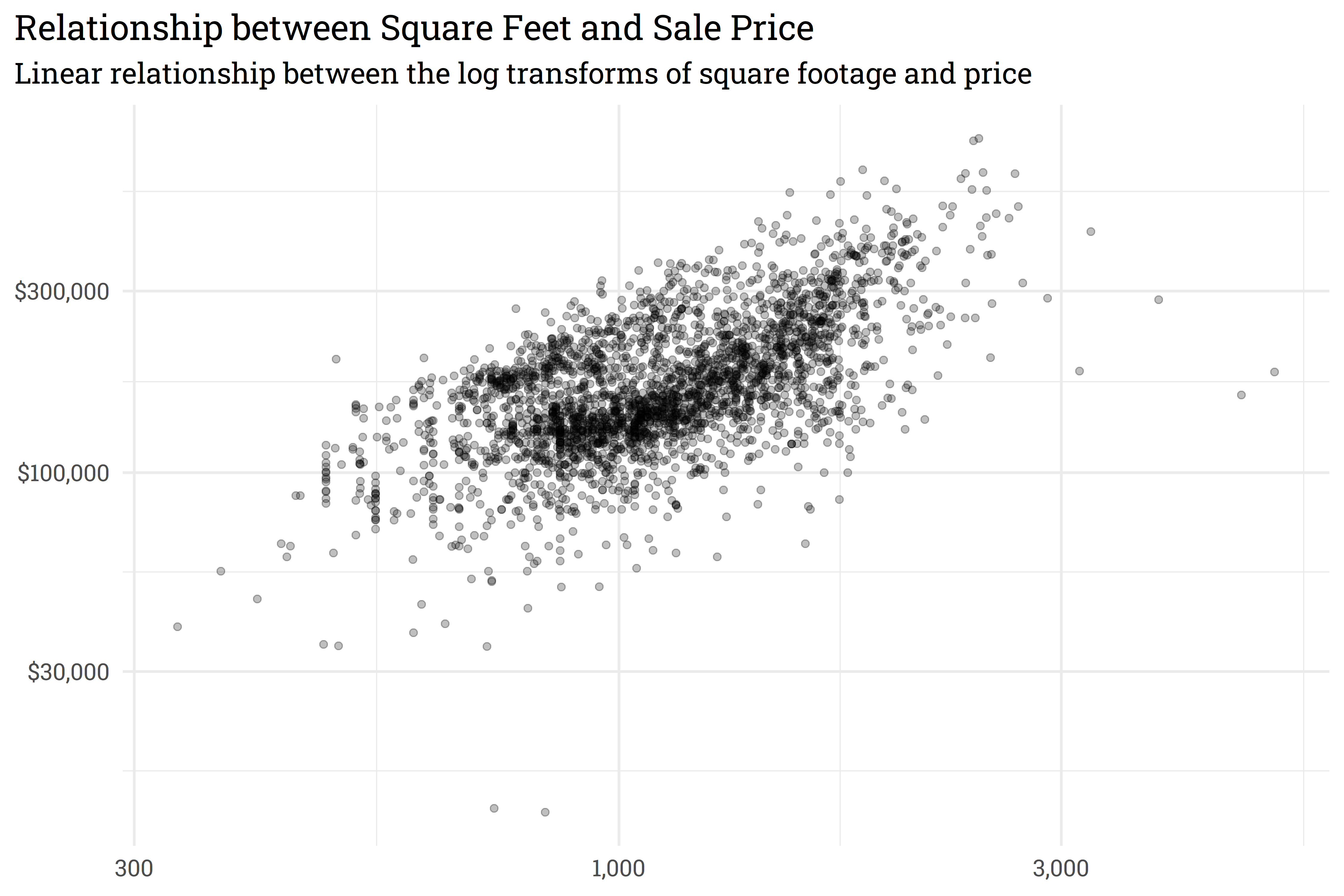We can use a linear model to predict the log transform of `Sale_Price` based on the log transform of `First_Flr_SF`. In this example, we’ll train a linear model then plot our predictions against a holdout set with a prediction interval.

Code
``````# log transform
ames_mod <-
ames_mod %>%
mutate(across(everything(), log10))

# split into train/test data
set.seed(918)
ames_split <- initial_split(ames_mod)
ames_train <- training(ames_split)
ames_test <- testing(ames_split)``````
Code
``````# train a linear model
set.seed(314)
mod <- lm(Sale_Price ~ First_Flr_SF, data = ames_train)

# predict on new data with a prediction interval
ames_preds <-
mod %>%
predict(ames_test, interval = "predict") %>%
as_tibble()``````
Code
``````# plot!
ames_preds %>%

# re-scale predictions to match the original dataset's scale
bind_cols(ames_test) %>%
mutate(across(everything(), ~10^.x)) %>%

ggplot(aes(x = First_Flr_SF)) +
geom_point(aes(y = Sale_Price),
alpha = 0.25) +
geom_line(aes(y = fit),
size = 1) +
geom_ribbon(aes(ymin = lwr,
ymax = upr),
alpha = 0.25) +
scale_y_continuous(labels = scales::dollar_format(), trans = "log10") +
scale_x_continuous(labels = scales::comma_format(), trans = "log10") +
labs(title = "Linear Model of Sale Price predicted by Square Footage",
subtitle = "Shaded area represents the 95% prediction interval",
x = NULL,
y = NULL) ``````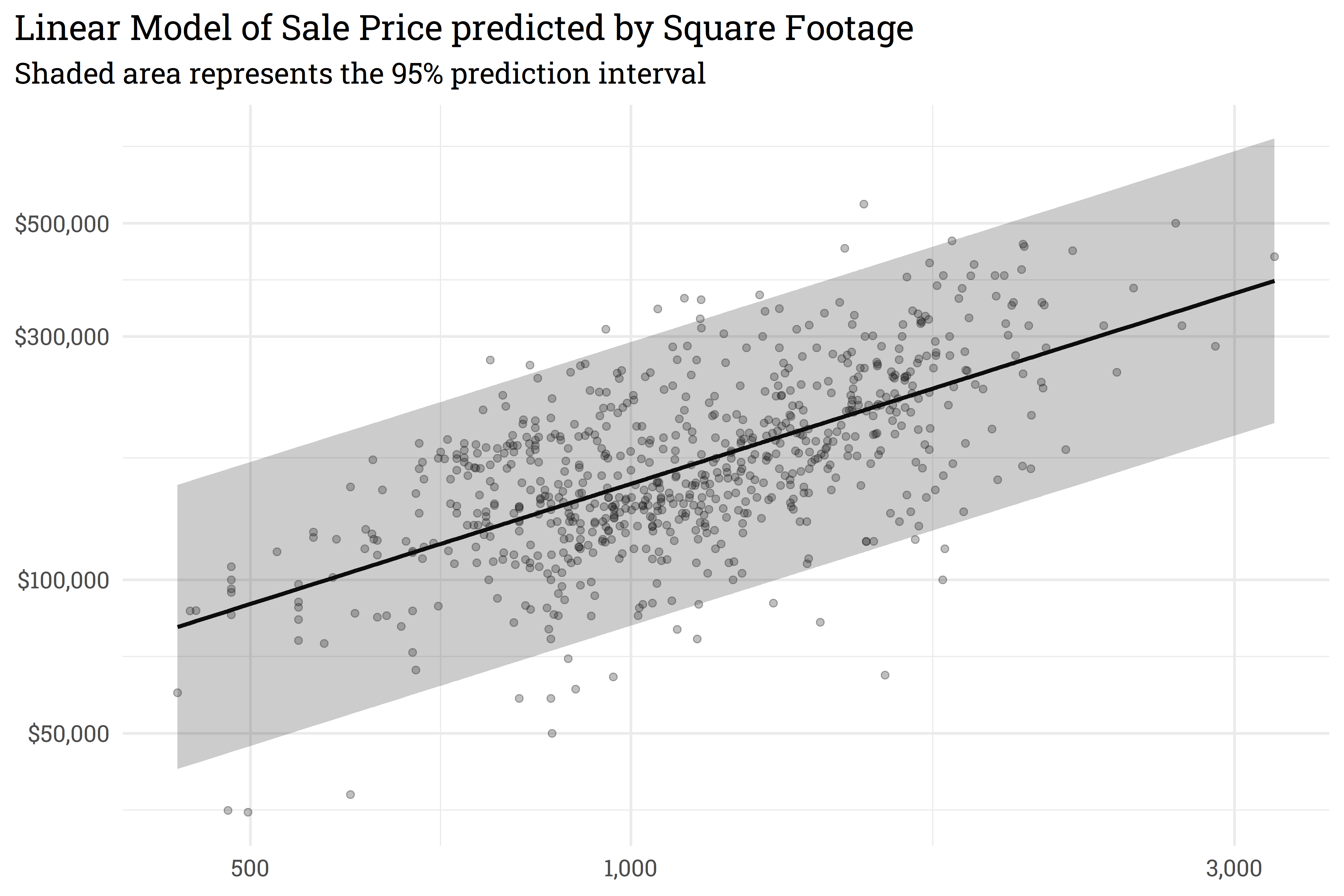With `{workboots}`, we can approximate the linear model’s prediction interval by passing a workflow built on a linear model to the function `predict_boots()`.

Code
``````library(tidymodels)
library(workboots)

# setup a workflow with a linear model
ames_wf <-
workflow() %>%
add_recipe(recipe(Sale_Price ~ First_Flr_SF, data = ames_train)) %>%

# generate bootstrap predictions on ames_test
set.seed(713)
ames_preds_boot <-
ames_wf %>%
predict_boots(
n = 2000,
training_data = ames_train,
new_data = ames_test
)``````

`predict_boots()` works by creating 2000 bootstrap resamples of the training data, fitting a linear model to each resample, then generating 2000 predictions for each home’s price in the holdout set. We can then use `summarise_predictions()` to generate upper and lower intervals for each prediction.

Code
``````ames_preds_boot %>%
summarise_predictions()``````
``````#> # A tibble: 733 × 5
#>    rowid .preds               .pred .pred_lower .pred_upper
#>    <int> <list>               <dbl>       <dbl>       <dbl>
#>  1     1 <tibble [2,000 × 2]>  5.44        5.17        5.71
#>  2     2 <tibble [2,000 × 2]>  5.27        4.98        5.55
#>  3     3 <tibble [2,000 × 2]>  5.25        4.97        5.52
#>  4     4 <tibble [2,000 × 2]>  5.40        5.11        5.67
#>  5     5 <tibble [2,000 × 2]>  5.44        5.15        5.71
#>  6     6 <tibble [2,000 × 2]>  5.21        4.93        5.49
#>  7     7 <tibble [2,000 × 2]>  4.94        4.67        5.22
#>  8     8 <tibble [2,000 × 2]>  5.13        4.85        5.40
#>  9     9 <tibble [2,000 × 2]>  5.14        4.87        5.42
#> 10    10 <tibble [2,000 × 2]>  5.41        5.13        5.69
#> # … with 723 more rows``````

By overlaying the intervals on top of one another, we can see that the prediction interval generated by `predict_boots()` is a good approximation of the theoretical interval generated by `lm()`.

Code
``````ames_preds_boot %>%
summarise_predictions() %>%
bind_cols(ames_preds) %>%
bind_cols(ames_test) %>%
mutate(across(c(.pred:Sale_Price), ~10^.x)) %>%
ggplot(aes(x = First_Flr_SF)) +
geom_point(aes(y = Sale_Price),
alpha = 0.25) +
geom_line(aes(y = fit),
size = 1) +
geom_ribbon(aes(ymin = lwr,
ymax = upr),
alpha = 0.25) +
geom_point(aes(y = .pred),
color = "blue",
alpha = 0.25) +
geom_errorbar(aes(ymin = .pred_lower,
ymax = .pred_upper),
color = "blue",
alpha = 0.25,
width = 0.0125) +
scale_y_continuous(labels = scales::dollar_format(), trans = "log10") +
scale_x_continuous(labels = scales::comma_format(), trans = "log10") +
labs(title = "Linear Model of Sale Price predicted by Square Footage",
subtitle = "Bootstrap prediction interval closely matches theoretical prediction interval",
x = NULL,
y = NULL)``````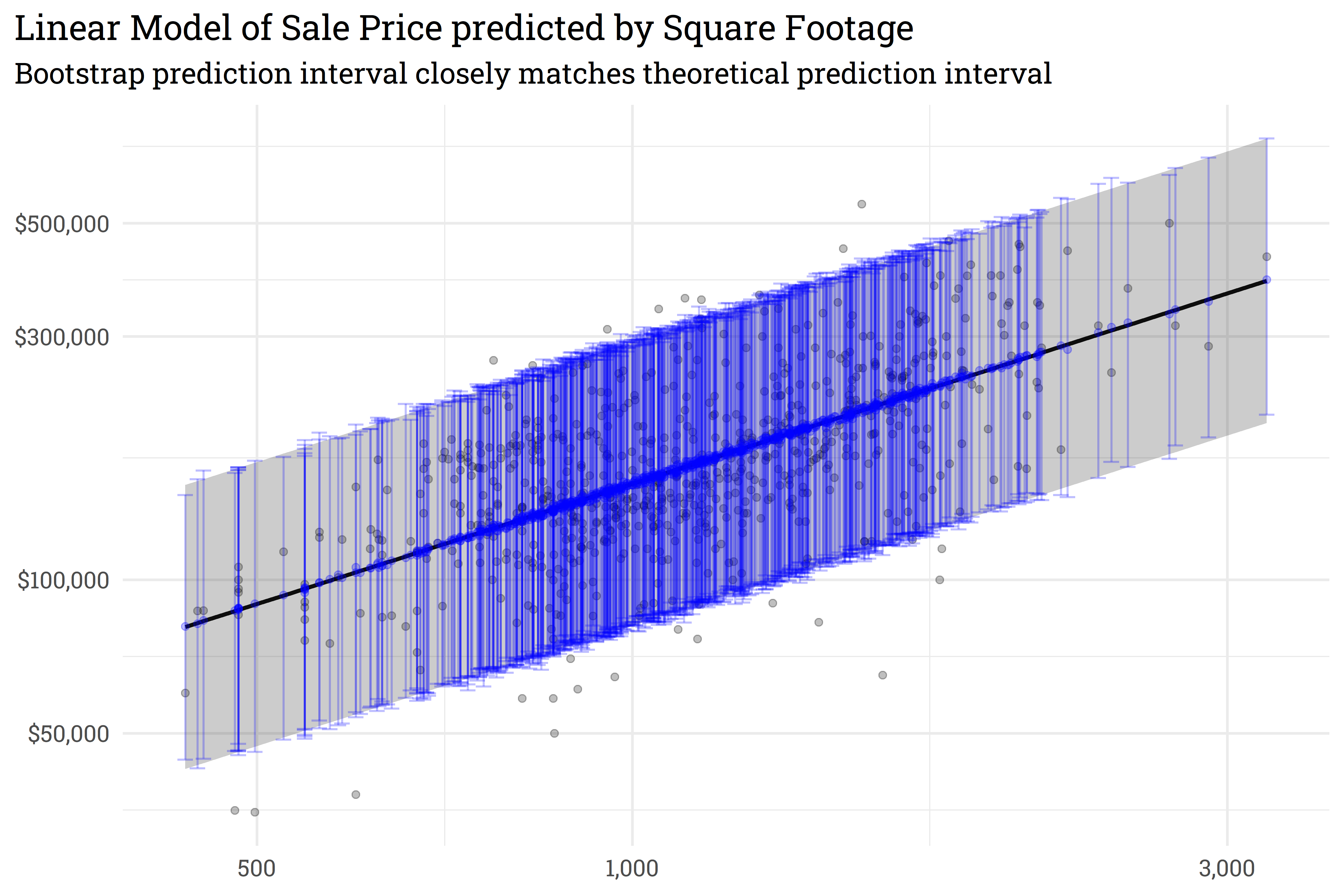Both `lm()` and `summarise_predictions()` use a 95% prediction interval by default but we can generate other intervals by passing different values to the parameter `conf`:

Code
``````ames_preds_boot %>%

# generate 95% prediction interval
summarise_predictions(conf = 0.95) %>%
rename(.pred_lower_95 = .pred_lower,
.pred_upper_95 = .pred_upper) %>%
select(-.pred) %>%

# generate 80% prediction interval
summarise_predictions(conf = 0.80) %>%
rename(.pred_lower_80 = .pred_lower,
.pred_upper_80 = .pred_upper) %>%
bind_cols(ames_test) %>%
mutate(across(c(.pred_lower_95:Sale_Price), ~10^.x)) %>%

# plot!
ggplot(aes(x = First_Flr_SF)) +
geom_point(aes(y = Sale_Price),
alpha = 0.25) +
geom_line(aes(y = .pred),
size = 1,
color = "blue") +
geom_ribbon(aes(ymin = .pred_lower_95,
ymax = .pred_upper_95),
alpha = 0.25,
fill = "blue") +
geom_ribbon(aes(ymin = .pred_lower_80,
ymax = .pred_upper_80),
alpha = 0.25,
fill = "blue") +
scale_y_continuous(labels = scales::dollar_format(), trans = "log10") +
scale_x_continuous(labels = scales::comma_format(), trans = "log10") +
labs(title = "Linear Model of Sale Price predicted by Square Footage",
subtitle = "Predictions alongside 95% and 80% bootstrap prediction interval",
x = NULL,
y = NULL)``````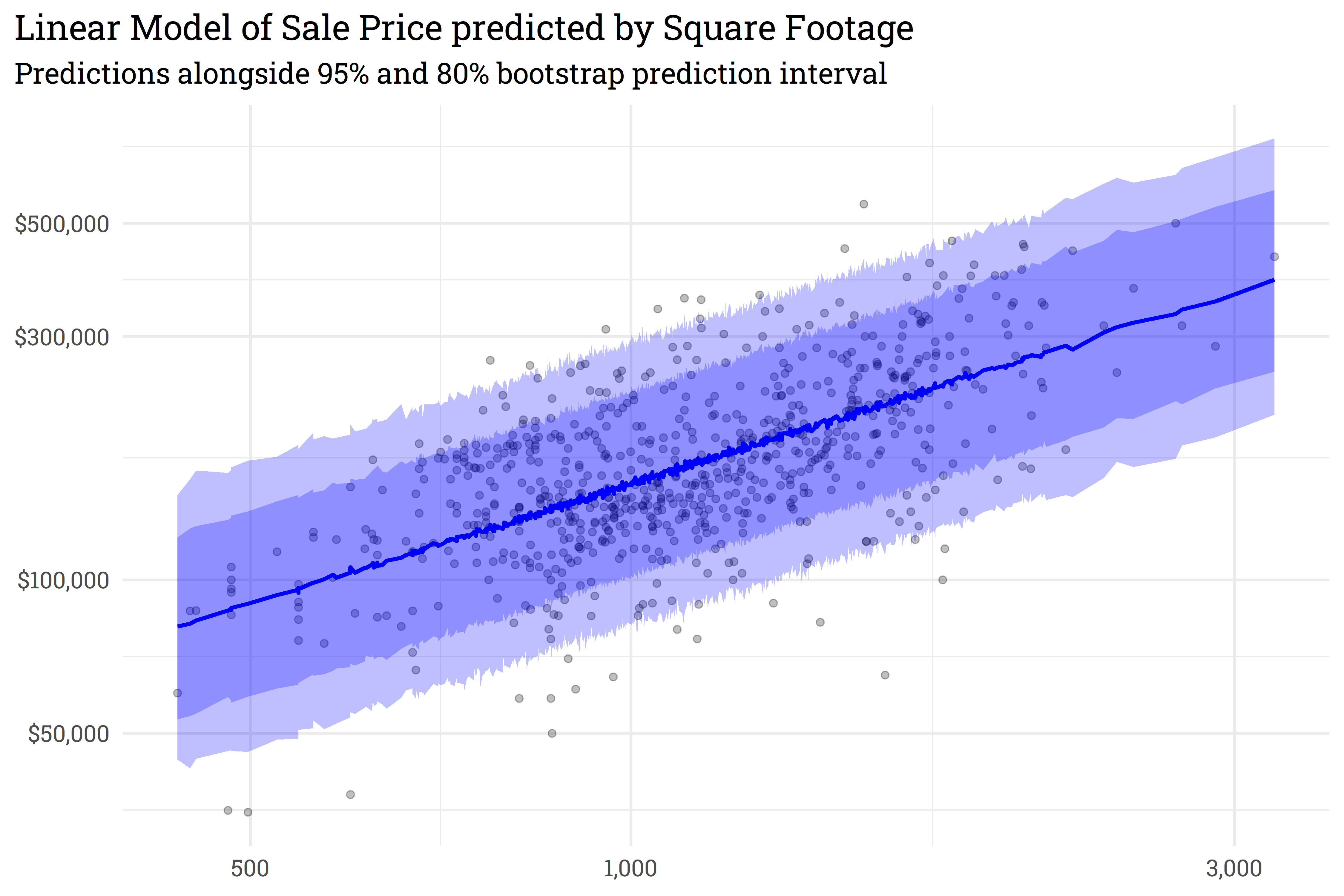As this example shows, `{workboots}` can approximate linear prediction intervals pretty well! But this isn’t very useful, since we can just generate a linear prediction interval from a linear model directly. The real benefit of `{workboots}` comes from generating prediction intervals from any model!

## Bootstrap prediction intervals with non-linear models

XGBoost is one of my favorite models. Up until now, however, in situations that require a prediction interval, I’ve had to opt for a simpler model. With `{workboots}`, that’s no longer an issue! In this example, we’ll use XGBoost and `{workboots}` to generate predictions of a penguins weight from the Palmer Penguins dataset.

To get started, let’s build a workflow and train an individual model.

Code
``````# load and prep data
data("penguins")

penguins <-
penguins %>%
drop_na()

# split data into training and testing sets
set.seed(123)
penguins_split <- initial_split(penguins)
penguins_test <- testing(penguins_split)
penguins_train <- training(penguins_split)``````
Code
``````# create a workflow
penguins_wf <-
workflow() %>%

recipe(body_mass_g ~ ., data = penguins_train) %>%
step_dummy(all_nominal_predictors())
) %>%

boost_tree("regression")
)

# fit to training data & predict on test data
set.seed(234)
penguins_preds <-
penguins_wf %>%
fit(penguins_train) %>%
predict(penguins_test)``````

As mentioned above, XGBoost models can only generate point predictions.

Code
``````penguins_preds %>%
bind_cols(penguins_test) %>%
ggplot(aes(x = body_mass_g,
y = .pred)) +
geom_point() +
geom_abline(linetype = "dashed",
color = "gray") +
labs(title = "XGBoost Model of Penguin Weight",
subtitle = "Individual model can only output individual predictions")``````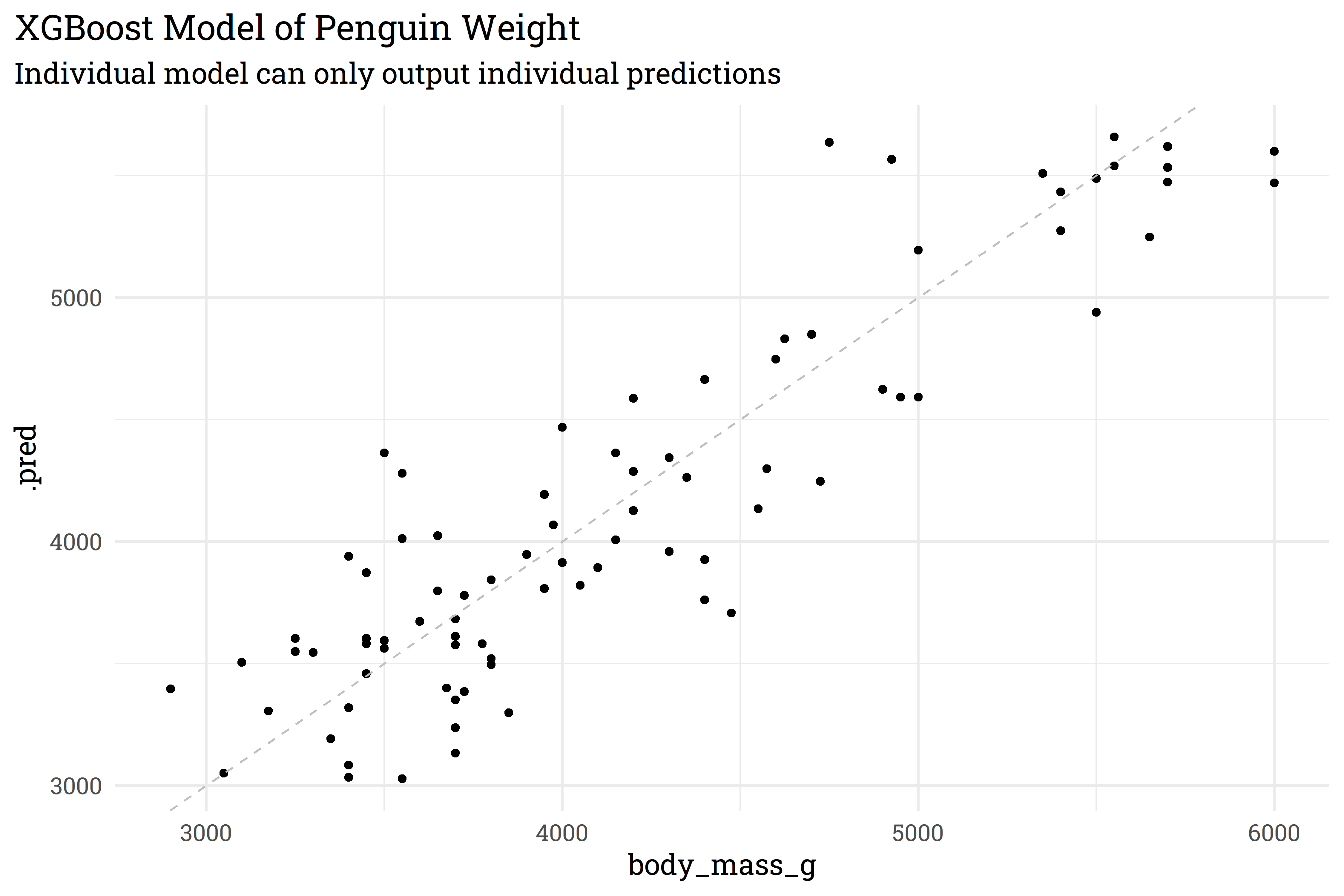With `{workboots}`, however, we can generate a prediction interval from our XGBoost model for each penguin’s weight!

Code
``````# create 2000 models from bootstrap resamples and make predictions on the test set
set.seed(345)
penguins_preds_boot <-
penguins_wf %>%
predict_boots(
n = 2000,
training_data = penguins_train,
new_data = penguins_test
)

penguins_preds_boot %>%
summarise_predictions()``````

How does our bootstrap model perform?

Code
``````penguins_preds_boot %>%
summarise_predictions() %>%
bind_cols(penguins_test) %>%
ggplot(aes(x = body_mass_g,
y = .pred,
ymin = .pred_lower,
ymax = .pred_upper)) +
geom_abline(linetype = "dashed",
color = "gray") +
geom_errorbar(alpha = 0.5,
color = "blue") +
geom_point(alpha = 0.5,
color = "blue") +
labs(title = "XGBoost Model of Penguin Weight",
subtitle = "Bootstrap models can generate prediction intervals")``````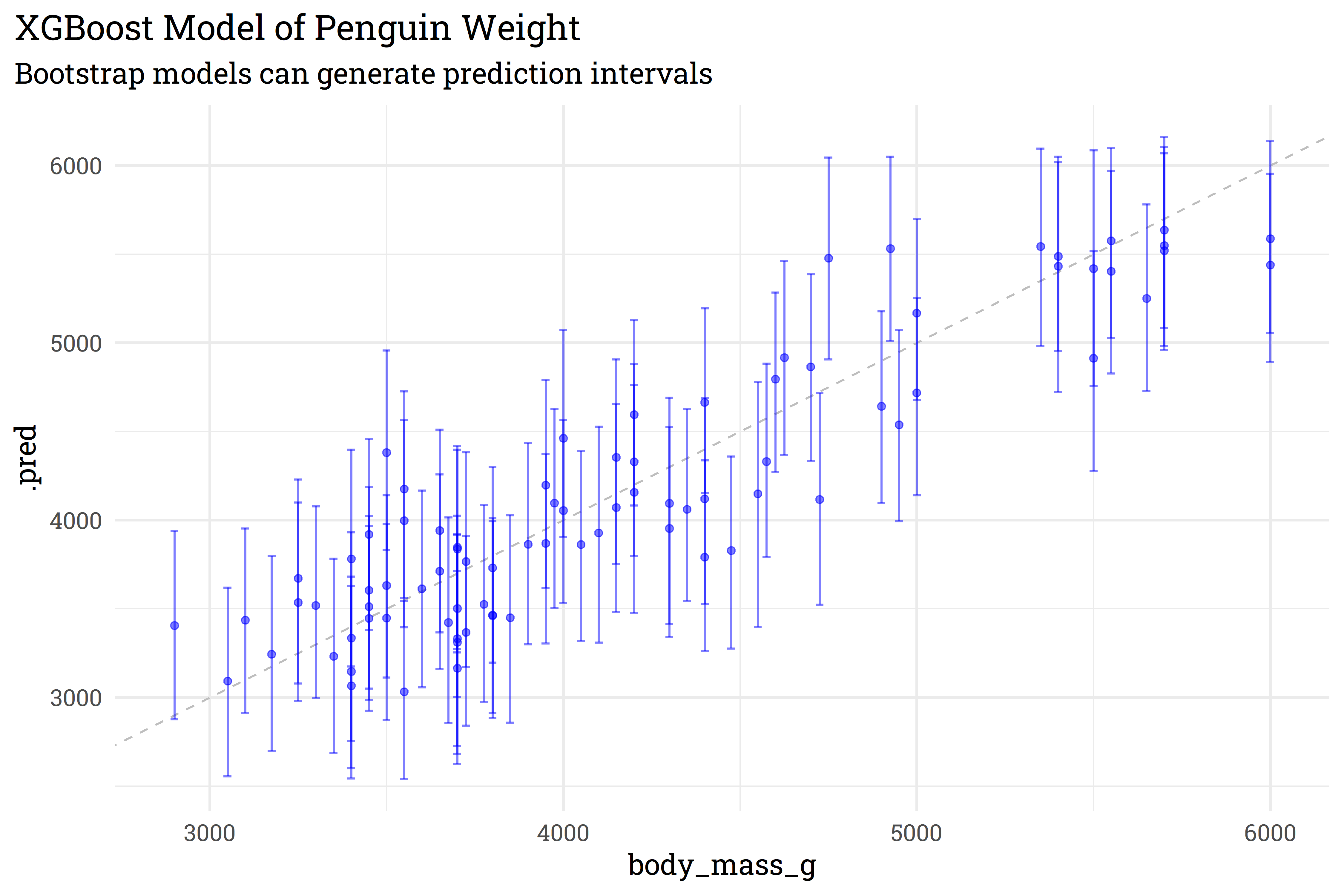This particular model may be in need of some tuning for better performance, but the important takeaway is that we were able to generate a prediction distribution for the model! This method works with other regression models as well — just create a workflow then let `{workboots}` take care of the rest!

# Tidymodel Resources

## Citation

BibTeX citation:
``````@online{rieke2022,
author = {Mark Rieke},
title = {Introducing \{Workboots\}},
date = {2022-03-14},